The MODEL Procedure

The Chow test is used to test for break points or structural changes in a model. The problem is posed as a partitioning of the data into two parts of size
and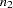. The null hypothesis to be tested is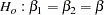where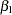is estimated by using the first part of the data and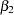is estimated by using the second part.

The test is performed as follows (see Davidson and MacKinnon 1993, p. 380).

1. The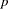parameters of the model are estimated.

2. A second linear regression is performed on the residuals,, from the nonlinear estimation in step one.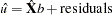where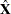is Jacobian columns that are evaluated at the parameter estimates. If the estimation is an instrumental variables estimation with matrix of instruments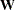, then the following regression is performed: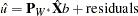where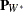is the projection matrix.

3. The restricted SSE (RSSE) from this regression is obtained. An SSE for each subsample is then obtained by using the same linear regression.

4. The F statistic is then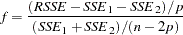This test hasand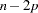degrees of freedom.

Chow’s test is not applicable if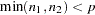, since one of the two subsamples does not contain enough data to estimate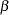. In this instance, the predictive Chow test can be used. The predictive Chow test is defined as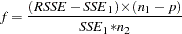where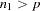. This test can be derived from the Chow test by noting that the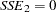when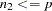and by adjusting the degrees of freedom appropriately.

You can select the Chow test and the predictive Chow test by specifying the CHOW=arg and the PCHOW=arg options in the FIT statement, where arg is either the number of observations in the first sample or a parenthesized list of first sample sizes. If the size of the one of the two groups in which the sample is partitioned is less than the number of parameters, then a predictive Chow test is automatically used. These tests statistics are not produced for GMM and FIML estimations.

The following is an example of the use of the Chow test.

```   data exp;
x=0;
do time=1 to 100;
if time=50 then x=1;
y = 35 * exp( 0.01 * time ) + rannor( 123 ) + x * 5;
output;
end;
run;

proc model data=exp;
parm zo 35 b;
dert.z = b * z;
y=z;
fit y init=(z=zo) / chow =(40 50 60) pchow=90;
run;
```

The data set introduces an artificial structural change into the model (the structural change effects the intercept parameter). The output from the requested Chow tests are shown in Figure 18.55.

Figure 18.55 Chow’s Test Results
The MODEL Procedure

Structural Change Test
Test Break Point Num DF Den DF F Value Pr > F
Chow 40 2 96 12.95 <.0001
Chow 50 2 96 101.37 <.0001
Chow 60 2 96 26.43 <.0001
Predictive Chow 90 11 87 1.86 0.0566Previous Page | Next Page | Top of Page